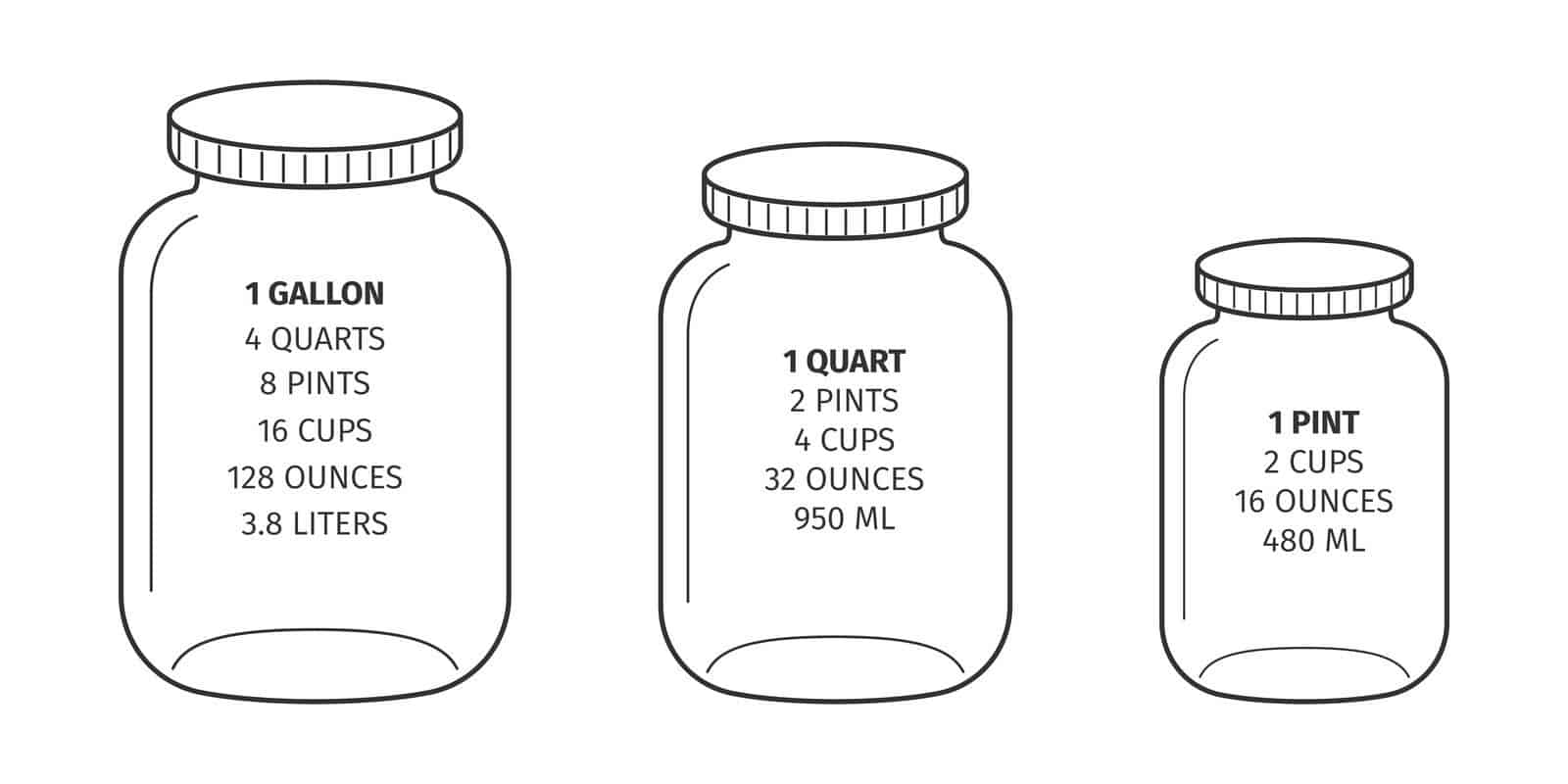# Understanding How Many Pints are in a QuartUnderstanding the capacity of a quart and the amount of pints it contains is important in the culinary world. This article will explain in detail the answer to the question “How many pints are in a quart?” We will examine the capacity of a quart, how it compares to other measuring units, and the conversion of a quart to pints.

## What is a Quart?

A quart is a unit of measurement that is used to measure volume and capacity. It is commonly used in the culinary world and is often used to measure liquid ingredients such as milk, water, and oil. A quart is a unit of measurement that is equal to two pints, or four cups. Quarts are typically measured in fluid ounces and are usually represented by the symbol “qt.”

## Comparing Quarts to Other Measuring Units

Quarts are often compared to other measuring units such as gallons, pints, and cups. A gallon is equal to four quarts, while a pint is equal to half a quart. A cup is equal to one-fourth of a quart. It is important to note that the amount of a quart can vary slightly depending on the type of liquid being measured.

## Converting Quarts to Pints

When converting quarts to pints, it is important to remember that one quart is equal to two pints. This means that if you have one quart of liquid, it will equal two pints. To convert quarts to pints, simply multiply the number of quarts by two. For example, if you have two quarts of liquid, it will equal four pints.

## Conclusion

In conclusion, understanding how many pints are in a quart is important in the culinary world. A quart is a unit of measurement that is equal to two pints, or four cups. When converting quarts to pints, simply multiply the number of quarts by two. Understanding the capacity of a quart and the amount of pints it contains can help cooks and bakers measure ingredients accurately.